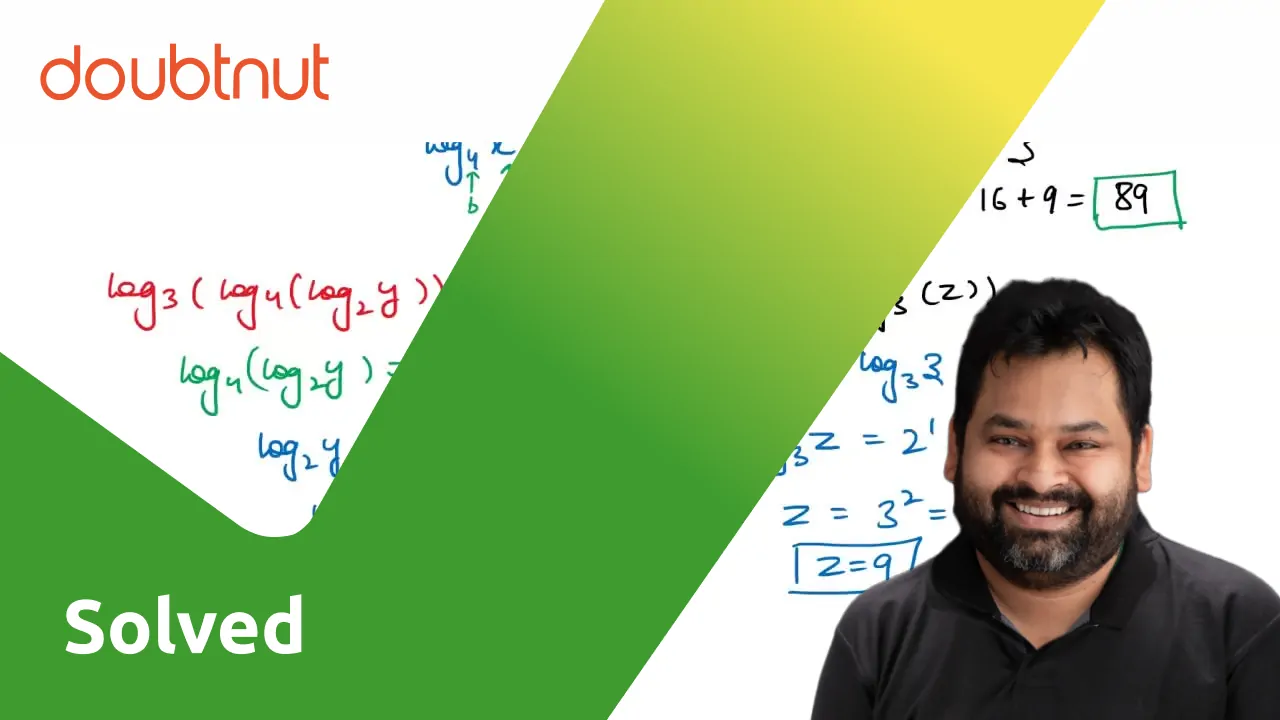Doubtnut App - Get 50 Lakh+ video solutions better than GoogleInstall Now

# If log2(log3(log4(x)))=0,log3(log4(log2(y)))=0 and log4(log2(log3(z)))=0 then the sum of x,y,z is

Video Solution